# Squirrel Cage Motors – Starting Methods & Speed Control System

## Starting Of Induction Motors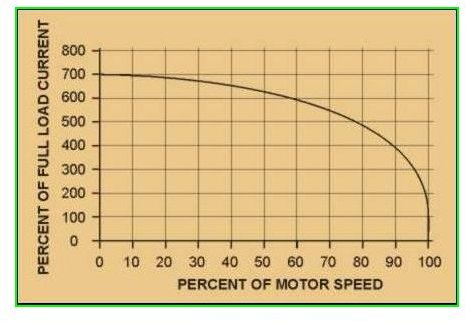It is already discussed in induction motors working principle that the rotor can be assumed as a rotating transformer. But the rotor of the induction motor has a short circuited secondary of the transformer. Therefore, if normal supply voltage is applied to a stationary motor, then similar to a transformer, a very large initial current is taken by the primary, for a short period of time. This is because; during starting there is no back e.m.f, to oppose the initial rush-in of the current. So, Induction motors when connected “direct on-line”, draw 5 to 7 times the original full load current. At this time, they develop only 1.5 to 2.5 times the full-load torque. This sudden initial drawing of current by an induction motor leads to heavy undesirable voltage dip which affects the other electrical machineries. Hence, any induction motor, whose rating above 25 kW to 40 kW should not be started direct on-line.

So what are the other methods of starting an Squirrel Cage Induction Motor?

They are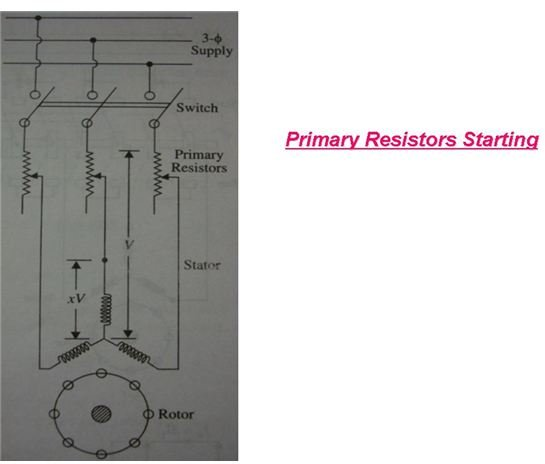1. Primary resistors (or rheostat) or reactors,

2. Auto-transformer or auto starter,

3. Star-Delta switches.

1. Primary Resistors: These resistors drop some voltage and hence reduce the voltage applied across the motor terminals. Thus the initial current drawn by the motor is reduced. This method is useful in starting only small motors because when the applied voltage across the motor terminals is reduced by 50%, then the current drawn by the motor also reduces by 50%, but the torque is reduced to 25% of the full-load value. Thus this method is used only for starting small motors.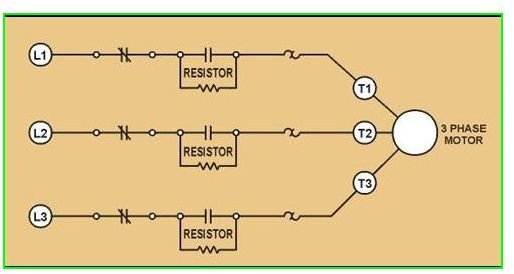2. Auto-Transformers: These are other-wise called as “auto-starters” or “compensators”, consists of auto-transformer with necessary switches. With necessary connections and switches, the voltage applied across the motor terminals can be varied or reduced till the motor reaches 80% of its full rated speed. Once the motor reaches its full rated speed, then just by interchanging switches, the full supply voltage is applied across the motor terminals. For small motors, the interchanging switches may be an “air switch”, but for bigger motors the switch is usually immersed inside oil as to prevent sparking. The motor protective devices like over-load and no-voltage protection can be incorporated in the circuit.

3. Star-Delta Starters: This starter is used if the motor is designed to run in delta-connected stator winding. This starter consists of two-way switch, which can be changed from star to delta while running. At start, the winding is star connected, so that the applied voltage over each phase of stator winding is reduced b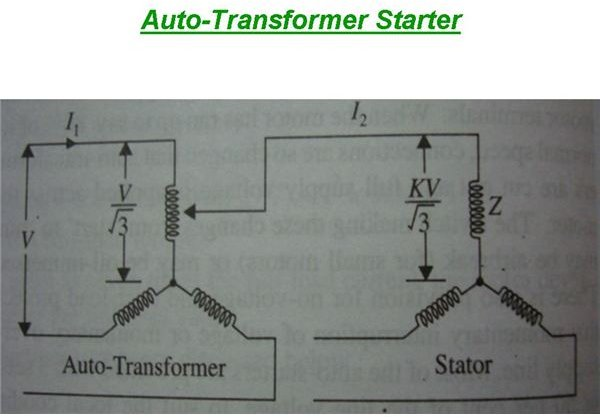y a factor 1/√3 and hence the torque developed becomes 1/3 of the torque that is developed if the motor is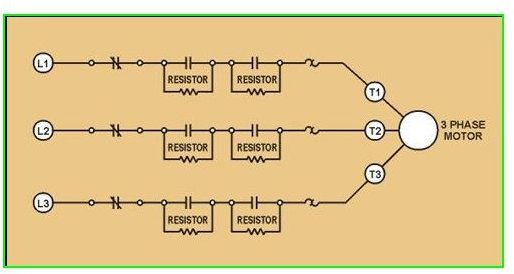directly delta connected. Thus the line current is reduced to 1/3. Thus during starting, the star connected motor, takes only 1/3 of the starting current and develops 1/3rd as much torque when connected directly in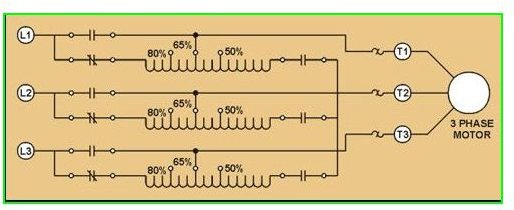delta.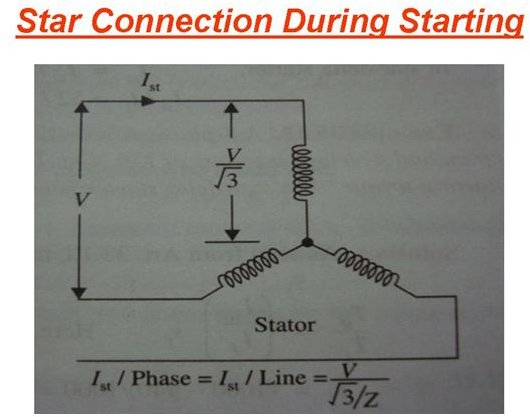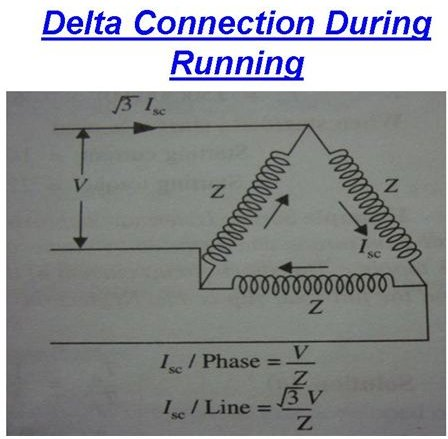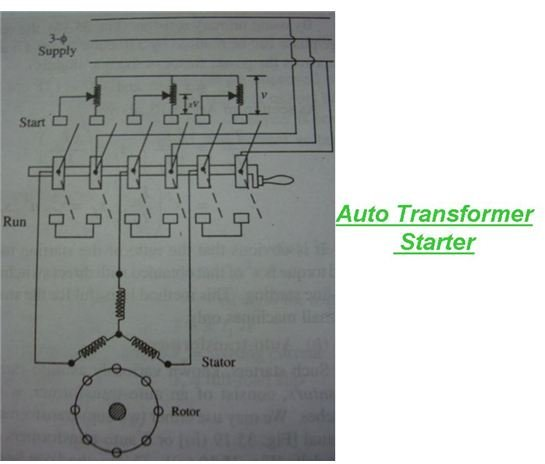There are also other methods of starting like "electronic" soft start arrangement and the split winding method.

## Speed Control of Induction Motors:

Unlike D.C. Motors, A.C. Induction Motors are not suitable for variable speeds. Their speed control and regulation is comparatively difficult when compared with D.C. Motors. These are some of the methods which are commonly used for the speed control of squirrel cage induction motors:

1. Changing Applied Voltage

2. Changing Applied Frequency

3. Changing Number Of Stator Poles

The above three methods are most commonly used for the speed control of squirrel cage Induction motors.

1. Changing Applied Voltage: This method, even though easiest, it is rarely used. The reasons are (a) for a small change in speed, there must be a large variation in voltage. (b) This large change in voltage will result in large change in flux density, thereby seriously disturbing the magnetic distribution/condition of the motor.

2. Changing Applied Frequency: We all know that the synchronous speed of the induction motor is given by Ns = 120f/P. So from this relation, it is evident that the synchronous speed and thus the speed of the induction motor can by varied by the supply frequency. This method has its own limitations. The motor speed can be reduced by reducing the frequency, if the induction motor happens to be the only load on the generators. Even then the range over which the speed can be varied is very less. This method is famous in some electrically driven ships although not common in shore.

3. Changing The Number Of Stator Poles: As we know the relation between the synchronous speed and the number of poles, i.e. Ns = 120f/P. So the number of poles is inversely proportional to the speed of the motor. This change of number of poles can be achieved by having two or more entirely independent stator windings in the same slots. Each winding gives a different number of poles and hence different synchronous speed. For example, for the same motor, if no. of poles = 2 , 4 or 6, which can be changed as per speed requirement, and lets say the supply frequency f = 50 Hz,

Then for

No. of Poles P = 2, then Ns = 120 * 50/2: So Ns = 3000 r.p.m

No. of Poles P = 4, then Ns = 120 * 50/4: So Ns = 1500 r.p.m

No. of Poles P = 6, then Ns = 120 * 50/6: So Ns = 1000 r.p.m.

Thus the speed control of squirrel cage induction motor can be done easily, but as steps of reduced speed. This method is used for elevator motors, traction motors and also for machine tools.

## Image & Content Credits

Clayton Engineering Website

A.C & D.C. Machines by Dr. Theraja